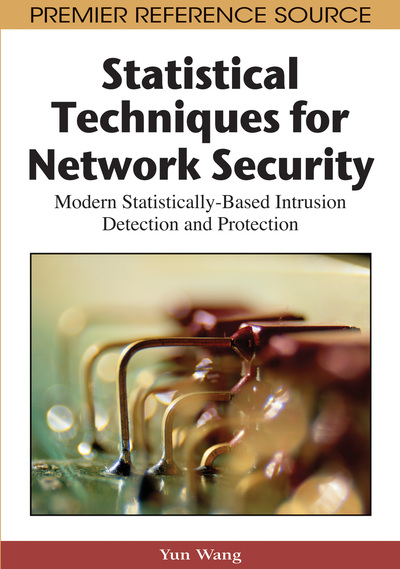# Network Data Characteristics

Yu Wang (Yale University, USA)
DOI: 10.4018/978-1-59904-708-9.ch004
Available
\$37.50
No Current Special Offers

## Abstract

Data represents the natural phenomena of our real world. Data is constructed by rows and columns; usually rows represent the observations and columns represent the variables. Observations, also called subjects, records, or data points, represent a phenomenon in the real world and variables, as also known as data elements or data fields, represent the characteristics of observations in data. Variables take different values for different observations, which can make observations independent of each other. Figure 4.1 illustrates a section of TCP/IP traffic data, in which the rows are individual network traffics, and the columns, separated by a space, are characteristics of the traffics. In this example, the first column is a session index of each connection and the second column is the date when the connection occurred. In this chapter, we will discuss some fundamental key features of variables and network data. We will present detailed discussions on variable characteristics and distributions in Sections Random Variables and Variables Distributions, and describe network data modules in Section Network Data Modules. The material covered in this chapter will help readers who do not have a solid background in this area gain an understanding of the basic concepts of variables and data. Additional information can be found from Introduction to the Practice of Statistics by Moore and McCabe (1998).
Chapter Preview

When you know you are doing your very best within the circumstances of your existence, applaud yourself!

— Rusty Berkus

Top

## Introduction

Data represents the natural phenomena of our real world. Data is constructed by rows and columns; usually rows represent the observations and columns represent the variables. Observations, also called subjects, records, or data points, represent a phenomenon in the real world and variables, as also known as data elements or data fields, represent the characteristics of observations in data. Variables take different values for different observations, which can make observations independent of each other. Figure 1 illustrates a section of TCP/IP traffic data, in which the rows are individual network traffics, and the columns, separated by a space, are characteristics of the traffics. In this example, the first column is a session index of each connection and the second column is the date when the connection occurred.

In this chapter, we will discuss some fundamental key features of variables and network data. We will present detailed discussions on variable characteristics and distributions in sections 4.2 and 4.3, and describe network data modules in section 4.4. The material covered in this chapter will help readers who do not have a solid background in this area gain an understanding of the basic concepts of variables and data. Additional information can be found from Introduction to the Practice of Statistics by Moore and McCabe (1998).

Top

## Random Variables

Understanding the concept of variables is important for developing and applying better statistical methods in network security. When we mention the word “variable”, we usually mean “random variable”—its value is a real number determined by each element of a sample space,(Stirzaker, 1999). A random variable can take on many possible values from the, but only one of those values will actually occur. For example, if we toss a coin five times and record each time the head faces up (true or false), the number of heads could take a value of 0, 1, 2, 3, 4 and 5, therefore, it is a random variable that is determined by the toss. Throughout this book we will use an uppercase letter,, to denote a random variable; its corresponding lowercase letter,, will denote its values. Most data elements in the network traffic are random variables. For example, ifis constructed by all possible destination IP addresses within a network, then an actual destination IP address of a randomly selected connection (i.e., every connection has the same chance to be selected) could be any one of the addresses in that sample space. Therefore, the variable that is used to represent the destination IP address is a random variable,. In Figure 4.1, however, the first column is not a random variable but an index to denote the order of sessions. For simplification, we will omit the term of “random” and only use the word “variable” throughout this book.

## Complete Chapter List

Search this Book:
Reset